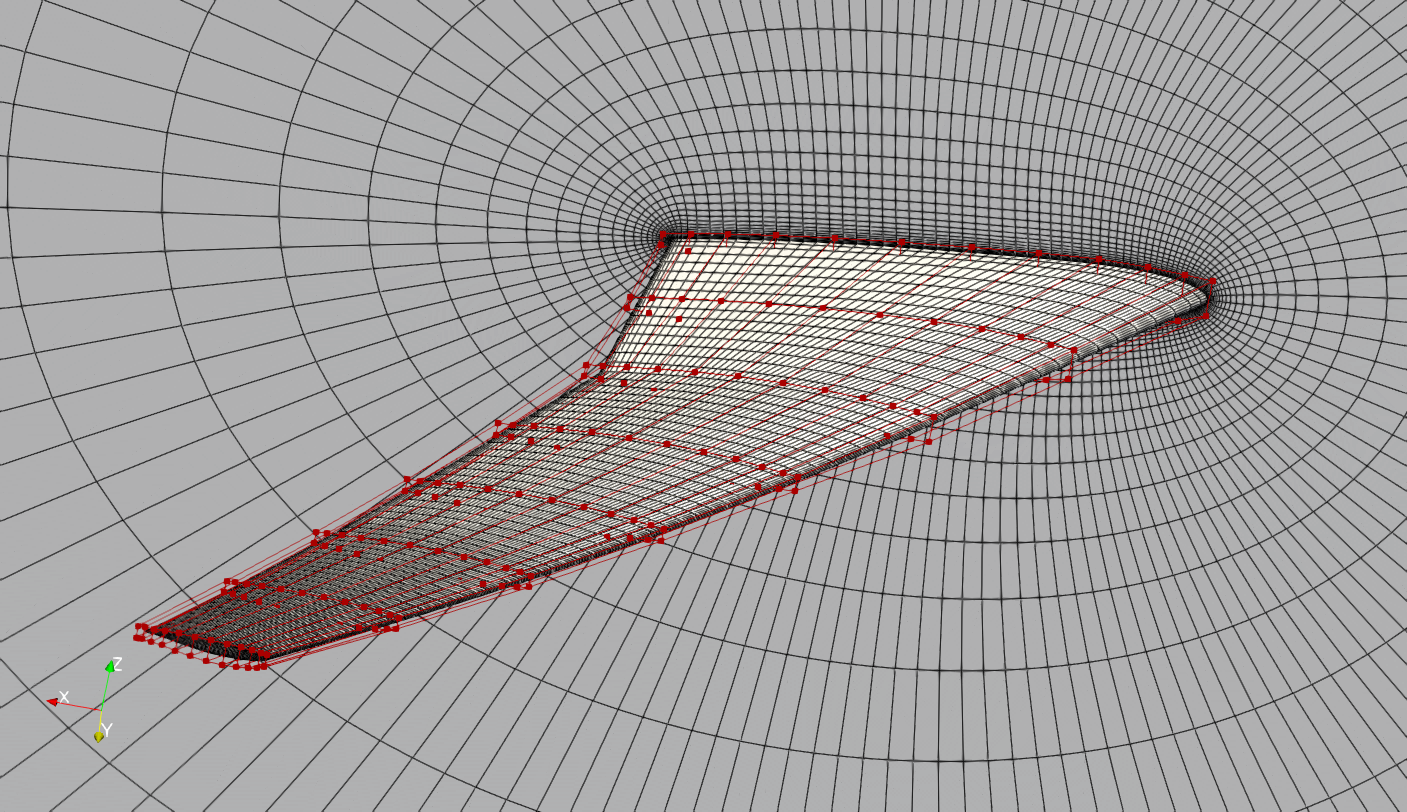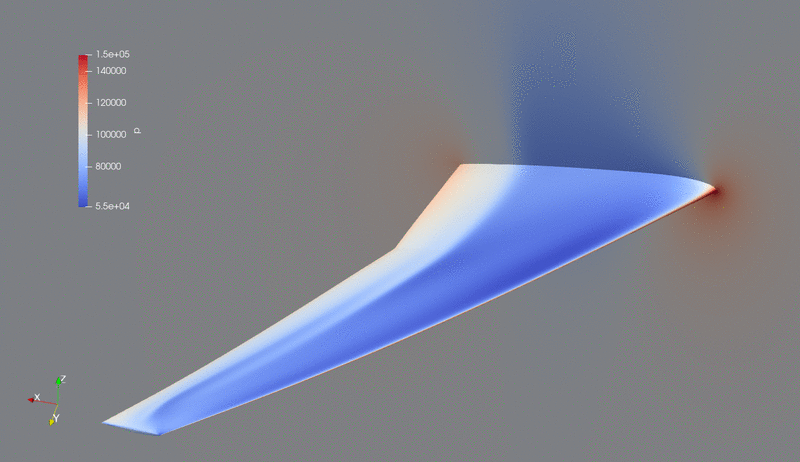The following is an aerodynamic shape optimization case for the common research model (CRM) wing at transonic condition.

```Case: Wing aerodynamic optimization
Geometry: CRM wing
Objective function: Drag coefficient (CD)
Lift coefficient (CL): 0.5
Design variables: 192 FFD points moving in the z direction, seven twists, and one angle of attack.
Constraints: volume, thickness, LE/TE, and lift constraints (total number: 768)
Mach number: 0.85
Reynolds number: 5 million
Mesh cells: ~579K
Solver: DARhoSimpleCFoam
```Fig. 1. Mesh and FFD points for the CRM wing.

The “runScript.py” is similar to that used in the Onera M6 wing.

To run this case, first download tutorials and untar it. Then go to tutorials-main/CRM_Wing and run the “preProcessing.sh” script to generate the mesh:

```./preProcessing.sh
```

Then, use the following command to run the optimization (we recommend using 64 cores):

```mpirun -np 64 python runScript.py 2>&1 | tee logOpt.txt
```

The case ran for 160 optimization iterations, the original CD was 0.02090 and the optimized CD was 0.01932 (7.6% drag reduction).

The evolution of pressure and shape during the optimization is as follows.Fig. 2. Pressure and shape evolution during the optimization process